There is no common form for spatial data that covers the complexity of geometric and topological types widely used in R. Traditional, GIS-alike spatial data is exceptional in the way that it does not make use of normal forms for geometric representations. We are working on a framework for complex, hierarchical data that can incorporate standard spatial types and allows working with them in a general and flexible way. We provide tools in R for more general representations of spatial primitives and the intermediate forms required for translation and analytical tasks.

The simple features standard has the following limitations:

• shapes are represented as paths so only planar polygonal shapes are possible
• the standard allows for `XY[Z[M]]` geometry,but this is not extensible - there is no capacity to store data against component geometry elements
• there is no capacity for internal topology (no vertex-, edge-, or path-sharing).

These limitations mean that it cannot represent in-full every-day objects from GPS, `rgl`, `ggplot2`/`ggvis`, `spatstat`, `maps`, TopoJSON, CAD drawings, or from 3D or general model structures. Translations between geo-spatial forms and the graphics and data grammars can be disjointed and sometimes awkward, relying on localized implementations that are lossy or inefficient, require 3rd party workflows, or involve unnecessary tasks.

`Simple features` is rightly seen as a corner-stone resource, but as a central basis for translations it is only able to handle a subset of the wider remit of “spatial data” in R. Topology in the form of component-element sharing (indexing of vertex, edge, arc, path) is not available to simple features, and while there are tools to generate it for certain planar cases, these are not explicitly available outside provided workflows and this information is not generally available for extensible uses.

Translation patterns that use simple features to translate data from native formats result in loss of information requiring complicated workarounds to preserve it. Common translations include in-memory structural representations, serialized forms within file formats, coordinate system geometry transformations, and topological or shape-modifying transformations.

## Illustration

This code generates a simple data set with the following properties:

• a data set of 2 POLYGONs
• the first polygon has one hole and one “concavity” (a three-vertex section of the path that differentiates the island from its convex hull)
• the second polygon is a single, convex island, and shares one edge with the first polygon
``````## two POLYGONs, composed of three rings p1+p2 and p3
p1 <- cbind(x = c(0, 0, 0.75, 1,   0.5, 0.8, 0.69, 0),
y = c(0, 1, 1,    0.8, 0.7, 0.6, 0,    0))
p2 <- cbind(x = c(0.2, 0.2, 0.3, 0.5, 0.5, 0.2),
y = c(0.2, 0.4, 0.6, 0.4, 0.2, 0.2))
p3 <- cbind(x = c(0.69, 0.8, 1.1, 1.23, 0.69),
y = c(0, 0.6, 0.63, 0.3, 0))
library(sf)
g <- data.frame(two_polygons = 1:2)
g[["geometry"]] <- st_sfc(st_polygon(list(p1, p2[nrow(p2):1, ])), st_polygon(list(p3)))
g <- st_as_sf(g)

## Delaunay triangulation of the polygon vertices, grouped by feature
gt <- g
st_geometry(gt)  <- st_triangulate(st_geometry(g))

op <- par(mfrow = c(1, 2))
plot(g)
plot(gt, col = scales::alpha(c("dodgerblue", "firebrick"), 0.3), main = "convex triangulation")
plot(st_geometry(g), add = TRUE, lwd = 4)``````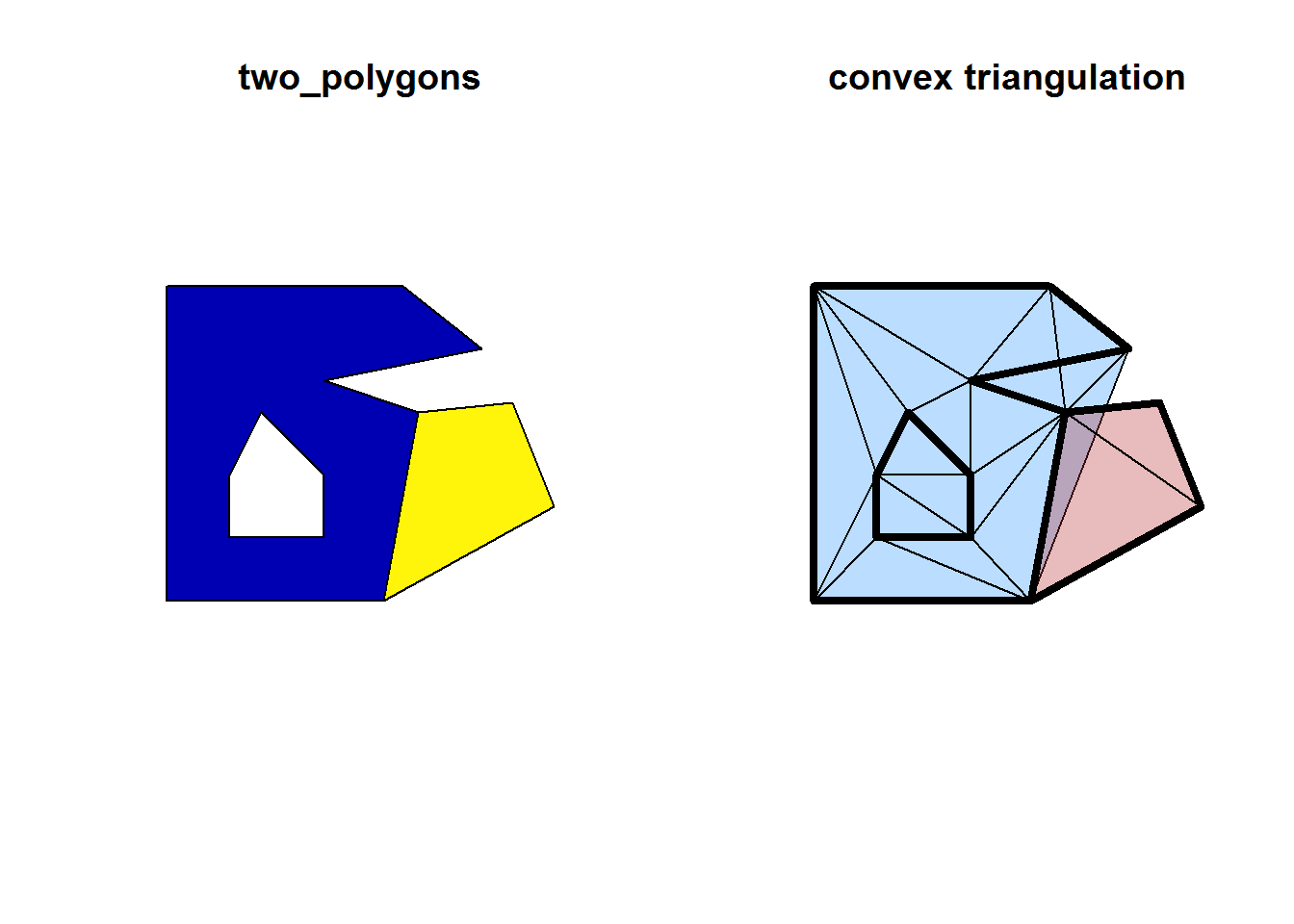``par(op)``

There is no capacity in the simple features standard to describe this situation in topological terms. The representation of these objects is always fully expanded, all instances of the vertices are stored explicitly with no record of duplications that close the rings within a polygon , or that represent shared coordinates between the two polygons. The relationship between the polygon island and its hole is unambiguous, but there is no identity of the hole or what it represents. There is no capacity to distinguish it from other parts of the polygon, and this is true of ever more complex objects with multiple islands, multiple holes, multiple connected lines and line segments.

Similarly in a MULTIPOINT there is no identity or grouping to each component vertex within the whole, and a single vertex has no information stored with it other than its position in an array and its raw geometric properties.

In the second panel is a Delaunay triangulation of the coordinates, grouped by feature. The triangles do not align with the polygon edges, because the triangle edges were not included in the triangulation algorithm. There is also an inserted overlap between the polygons, which makes this data set invalid for many standard GIS constructs. This is not a criticism of `st_triangulate` though, because even when we perform a constrained triangulation (Cheng et al., 2012), the simple features standard dictates that we store these all in TRIANGLE form, each a ring of four explicit coordinates within a GEOMETRYCOLLECTION. This can store the non-convex form of the triangulation, but only in fully expanded form - i.e. as a mesh with no indexing (http://postgis.net/docs/ST_GeometryN.html).

We can detect these properties using the in-built tools and return the exact relationship in object form. These tools internally build topological structure and then discard it.

``````## yes the first touches the second, and vice versa
sf::st_touches(g)``````
``````## []
##  2
##
## []
##  1``````
``````## each only covers itself, not the other
sf::st_covered_by(g)``````
``````## []
##  1
##
## []
##  2``````
``sf::st_crosses(g)``
``````## []
## integer(0)
##
## []
## integer(0)``````
``sf::st_intersects(g)``
``````## []
##  1 2
##
## []
##  1 2``````
``i <- sf::st_intersection(g[1, ], g[2, ])``
``````## Warning: attribute variables are assumed to be spatially constant
## throughout all geometries``````
``````plot(st_geometry(g))
plot(st_geometry(i), add = TRUE, lwd = 3, col = "dodgerblue")

op <- par(xpd = NA)
plot(st_geometry(st_cast(i, "MULTIPOINT")), add = TRUE, col = "firebrick",cex = 4)``````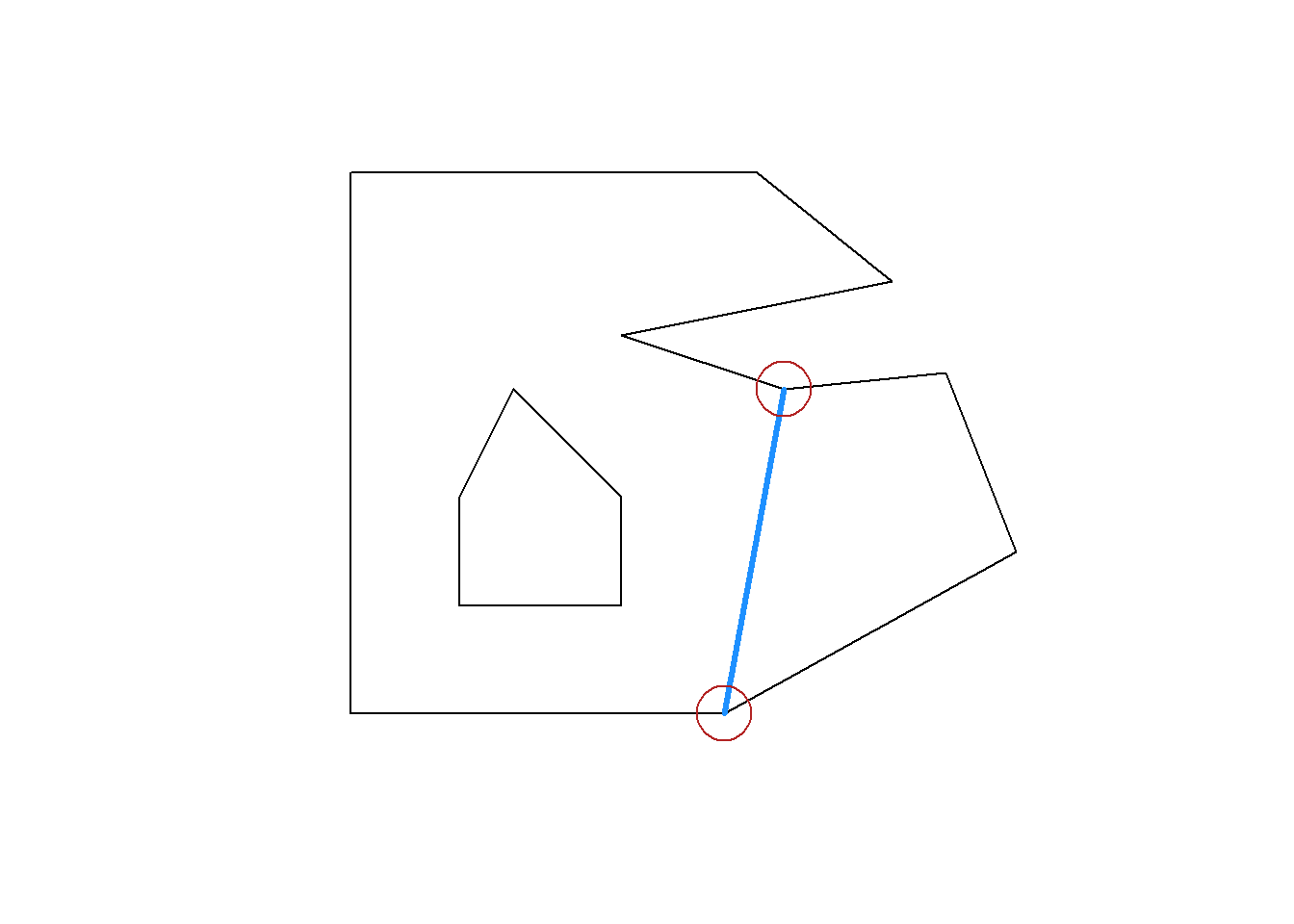``par(op)``

We got what we wanted, but how can we put this information back into the original object?

## Alternative representations

A path can be treated as a first-class type and and stored as such within a relational model, along with the other entities objects (“features”) and vertices. with this approach we gain two advantages, we can normalize the relations (detect and remove redundancy) and also store any additional data about the entitities in the model.

(There is an interplay between “able to store extra information” and “able to normalize”, since extra data may introduce further redundancy, but we defer this issue for now since full normalization is not our primary task).

An alternative form of representing this information is in the `sc` package, providing two main schemes, the `PATH` and the `PRIMITIVE` models. These provide different ways of linking objects and vertices. The two models are not mutually exclusive, and can co-exist sensibly within one data set. The PATH model can always be derived from the PRIMITIVE model and vice versa but the PRIMITIVE model has extra capacities that PATH cannot provide.

The key feature in `sc` is a relational model of indexed primitives and component elements. This provides a bridge to the traditionally structural, or array/matrix indexing and storage used in computer graphics and gaming.

The `PATH` models consists of three tables to store the objects (“features”), paths, and vertices, plus a fourth table `path_link_vertex` that allows de-duplication of the vertices (here in X-Y geometry).

``````#devtools::install_github("mdsumner/sc")
#devtools::install_github("mdsumner/scsf")
library(sc)
library(scsf)
## build the PATH model from a simple features object
str(sc::PATH(g))``````
``````## List of 4
##  \$ object          :Classes 'tbl_df', 'tbl' and 'data.frame':    2 obs. of  2 variables:
##   ..\$ two_polygons: int [1:2] 1 2
##   ..\$ object_     : chr [1:2] "4f9caa06" "319f3072"
##   ..- attr(*, "sf_column")= chr "geometry"
##   ..- attr(*, "agr")= Factor w/ 3 levels "constant","aggregate",..: NA
##   .. ..- attr(*, "names")= chr "two_polygons"
##  \$ path            :Classes 'tbl_df', 'tbl' and 'data.frame':    3 obs. of  3 variables:
##   ..\$ ncoords_: int [1:3] 8 6 5
##   ..\$ path_   : chr [1:3] "d225162a" "431ca458" "f05e7573"
##   ..\$ object_ : chr [1:3] "4f9caa06" "4f9caa06" "319f3072"
##  \$ vertex          :Classes 'tbl_df', 'tbl' and 'data.frame':    19 obs. of  3 variables:
##   ..\$ x_     : num [1:19] 0 0 0.75 1 0.5 0.8 0.69 0 0.2 0.5 ...
##   ..\$ y_     : num [1:19] 0 1 1 0.8 0.7 0.6 0 0 0.2 0.2 ...
##   ..\$ vertex_: chr [1:19] "a2821e22" "a2821e22" "a2821e22" "a2821e22" ...
##  \$ path_link_vertex:Classes 'tbl_df', 'tbl' and 'data.frame':    3 obs. of  2 variables:
##   ..\$ path_  : chr [1:3] "d225162a" "431ca458" "f05e7573"
##   ..\$ vertex_: chr [1:3] "a2821e22" "b893ca0d" "92b3fbff"
##  - attr(*, "class")= chr [1:2] "PATH" "sc"
##  - attr(*, "join_ramp")= chr [1:4] "object" "path" "path_link_vertex" "vertex"``````

The `PRIMITIVE` model is derived from and incorporates the `PATH` model, but adds 1-dimensional primitives (`segment`) to the collection of tables.

``````set.seed(39)
names(prim <- sc::PRIMITIVE(g))``````
``````##  "object"           "path"             "vertex"
``str(prim[c("segment")])``
``````## List of 1
##  \$ segment:Classes 'tbl_df', 'tbl' and 'data.frame': 16 obs. of  4 variables:
##   ..\$ .vertex0: chr [1:16] "1c324154" "1c324154" "1c324154" "1c324154" ...
##   ..\$ .vertex1: chr [1:16] "1c324154" "1c324154" "1c324154" "1c324154" ...
##   ..\$ path_   : chr [1:16] "3ed7380e" "3ed7380e" "3ed7380e" "3ed7380e" ...
##   ..\$ segment_: chr [1:16] "9ef7f4d8" "6438e89d" "e67be168" "60c3f8f0" ...``````

These representations provide a relational form stored in tables. This kind of data is usually stored as structural arrays, where the indexing is inherently related to the physical size of the vertex array (this is what an `rgl` object is). The `segment` table adds links to the first and second vertex of every line segment, and also records which `path` it belongs to. This is not the final design, since a segment can belong to multiple paths (albeit with a different orientation), and it is not clear how much one of these forms requires all of the path and primitive information together.

We can extract the topological information directly, because it’s actually stored explicitly within the data structures already.

``````library(dplyr)

get_shared_edge <- function(x, ...) UseMethod("get_shared_edge")
get_shared_edge.sf <- function(x, ...) {
prim <- sc::PRIMITIVE(x)
shared <- prim\$segment %>% dplyr::select(.vertex0, .vertex1) %>%
mutate_all(funs("I" = as.integer(factor(.))))  %>%
mutate(edge_id = paste(pmin(.vertex0_I, .vertex1_I), pmax(.vertex0_I, .vertex1_I), sep = "_")) %>%
group_by(edge_id) %>% filter(n() > 1) %>% ungroup() %>% select(.vertex0, .vertex1, edge_id)

## use this information to generate sf output
g0 <- shared %>% inner_join(prim\$vertex, c(".vertex0" = "vertex_")) %>% split(.\$edge_id) %>%
purrr::map(function(x) st_linestring(as.matrix(x %>% dplyr::select(x_, y_)))) %>% st_sfc()
st_sf(a = seq_along(g0), geometry = g0)
}

shared_e <- get_shared_edge(g)

plot(st_geometry(g))
plot(st_geometry(shared_e), add = TRUE, lwd = 3, col = "dodgerblue")

op <- par(xpd = NA)
plot(st_geometry(st_cast(shared_e, "MULTIPOINT")), add = TRUE, col = "firebrick",cex = 4)``````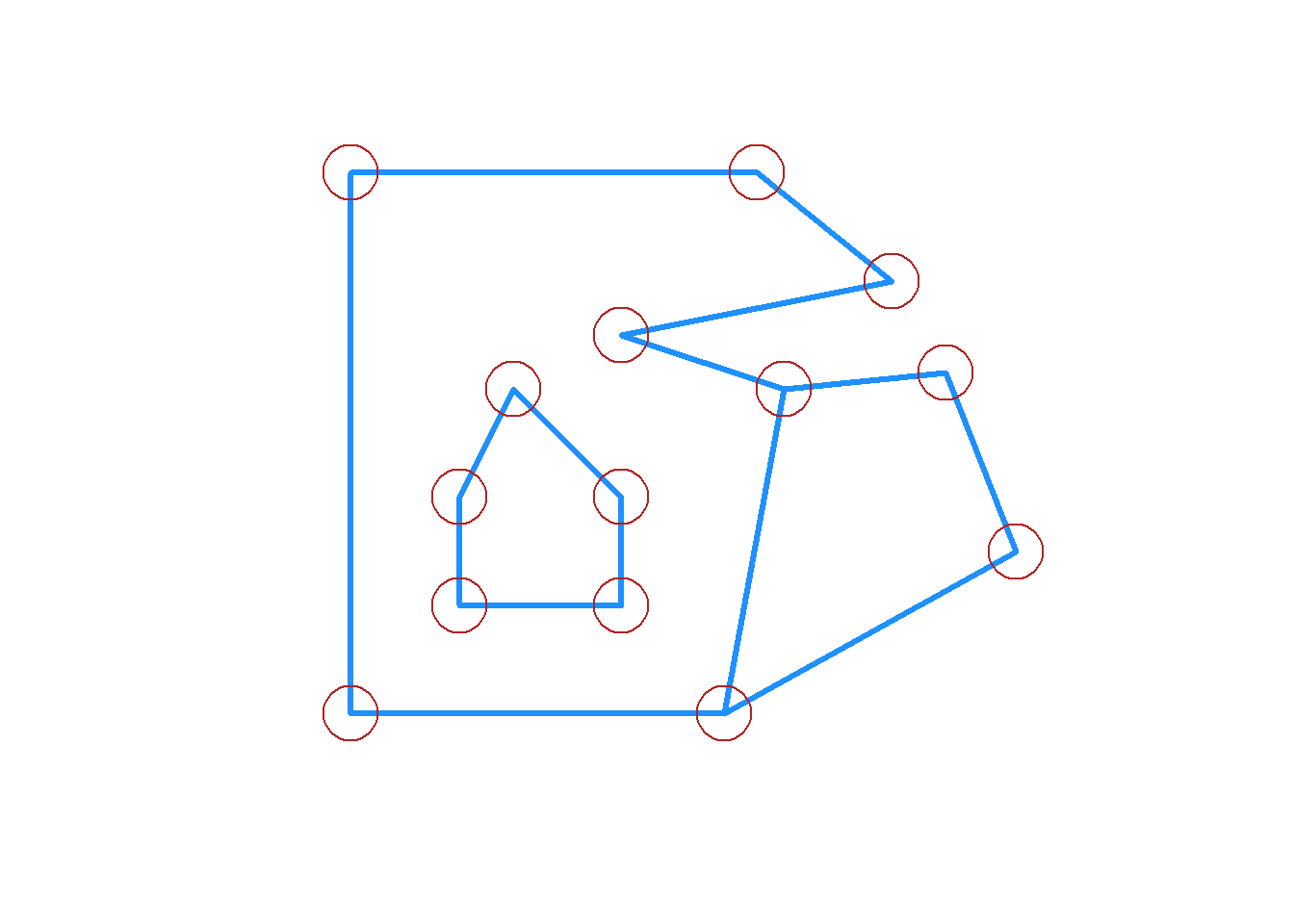``par(op)``

Apply this idea to a more complex data set.

``````nc <- st_read(system.file("shape/nc.shp", package="sf"), quiet = TRUE)

edge1 <- get_shared_edge(nc)
plot(st_geometry(nc), col = viridisLite::viridis(nrow(nc)))``````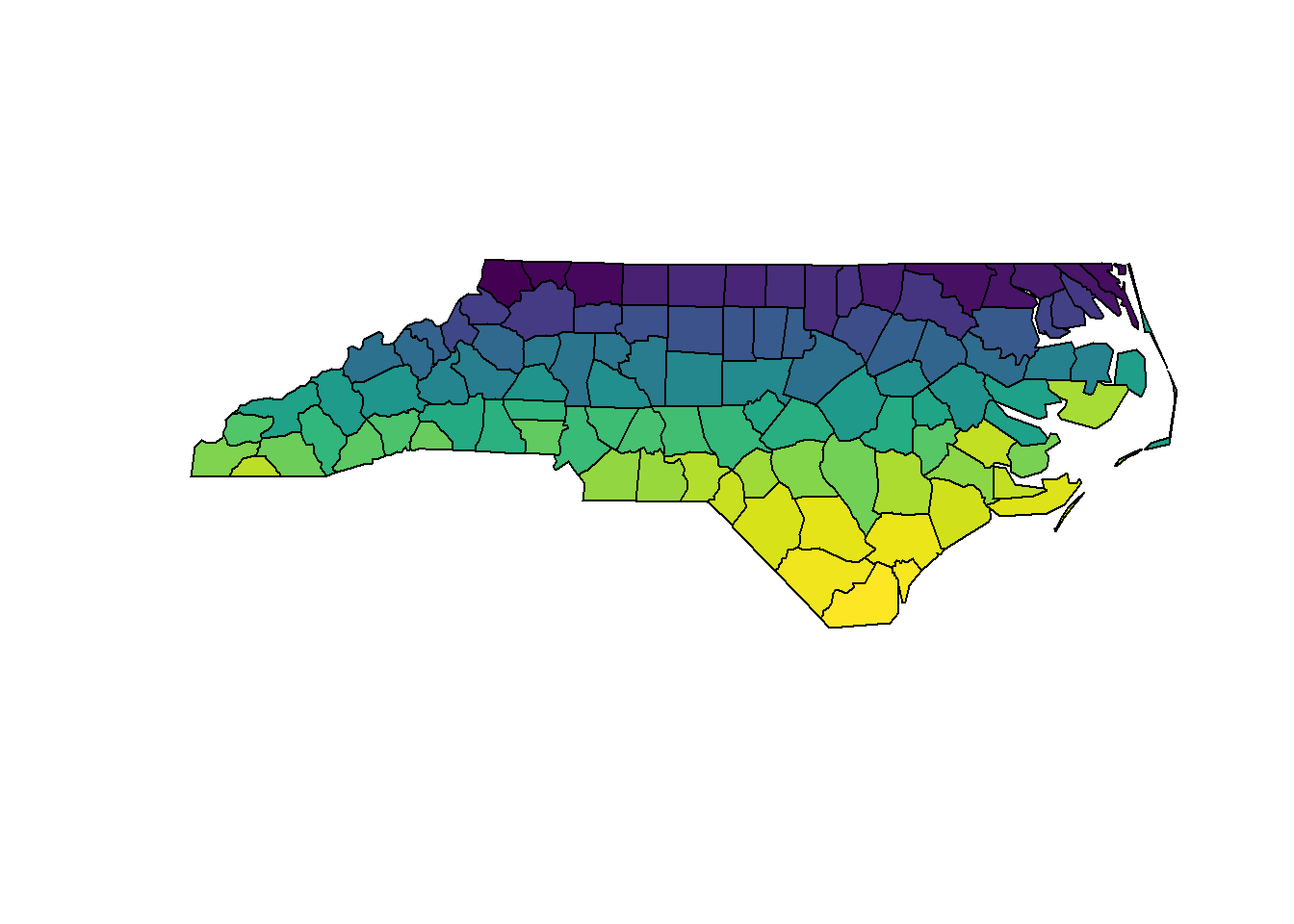``plot(st_geometry(edge1), col = viridisLite::inferno(nrow(edge1)), lwd = 2)``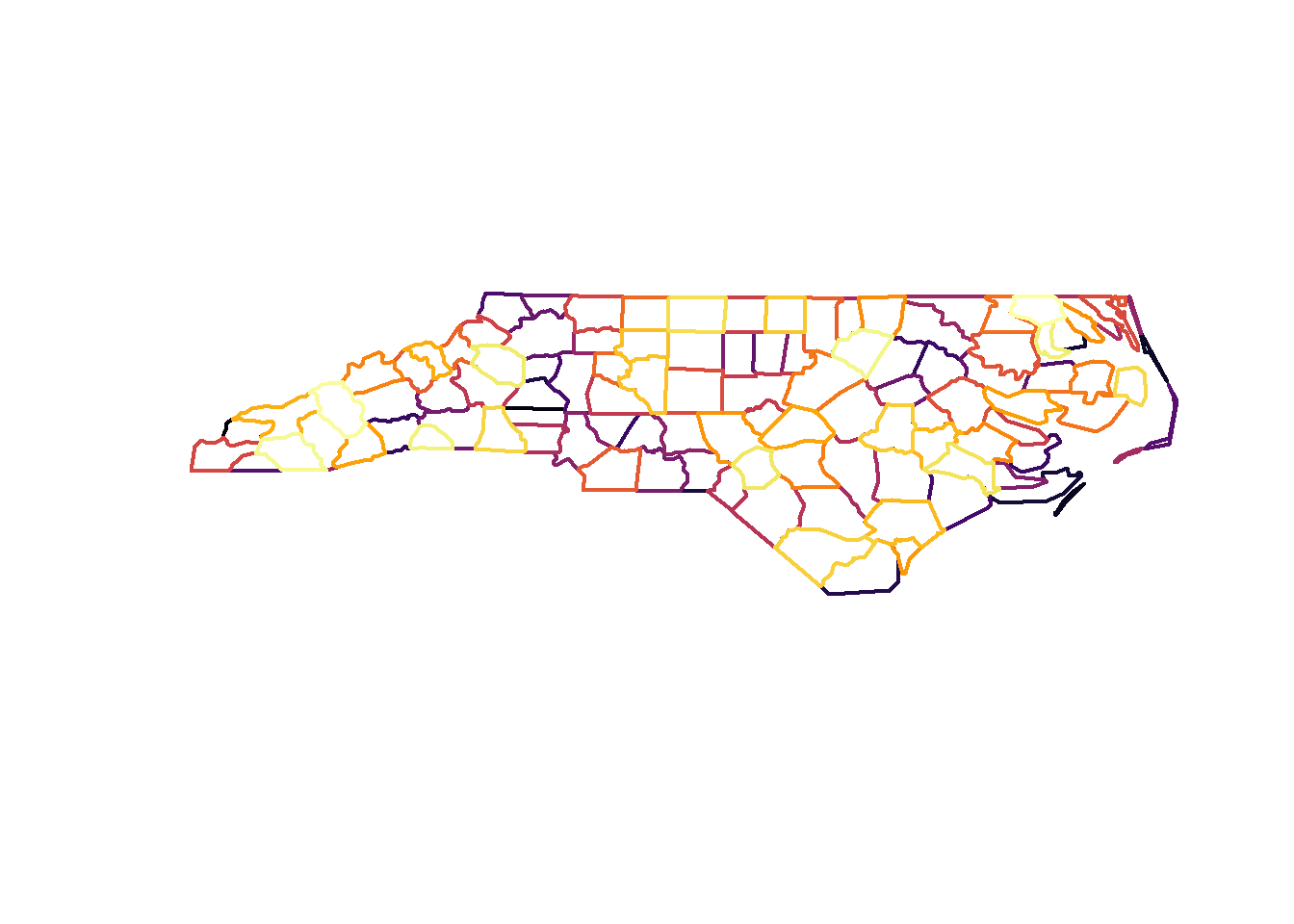The `edge1` data set is now an intermediate structure on the way to being an arc-node topology representation of all the shared arcs in this polygon layer. The implementation of this will avoid creating the simple features output however, as that is not able to natively store the index arc-paths.

Research the best ways forward

Investigate options for front-end user interfaces and back-end systems, and seek advice from key experts.

Implementations that we have considered include:

• lists of tables, as illustrated in proto-forms in spbabel, rangl, rbgm
• sf-like forms with nested list-columns of indexes, shared-entity semantics

and these raise the key issue that one data frame cannot store topological data where geometric elements are shared between features. Lists of tables are fine, and work well as a development idiom, but require some advanced design to find the right user-experience. We have erred on the side of unique indexes that allow arbitrary splitting and recombination of entities, but this is obviously expensive and requires work to only apply unique indexing when required, and otherwise use structural or matrix idioms for efficiencies.

Other options might include

• advanced programming techniques, using environments, R6, with vertex/primitives pools
• database or database-like connections in list-columns

Define language and classifications for the forms

The following entities underlie spatial objects: vertices, coordinates (instances of vertices), line segments, paths (linestrings, polygon rings, arcs), parts (rings, holes, linestrings, points), and objects or features.

We need relational models and the following broad components to a grammar of spatial data:

• a language of paths-belong-features, (a POINT can be a degenerate PATH for a MULTIPOINT) this incorporates TopoJSON, the internal `maps` store
• a language of vertices-belong-edges-belong-parts-belong-features, all sf is expressible with 1-D primitives, and this scheme incorporates GPS and other tracking-sensor data
• a language of vertices-belong-triangles-belong-parts-belong-features, which incorporates `rgl`, CAD drawings, 3D and general models.

Key outputs

1. Provide tools for decomposing geo-spatial and other complex data to common general forms, including topological indexing.
2. Illustrate general workflow with tools to convert between `sf`, GeoJSON, TopoJSON, leaflet list-forms, and `rgl` and `plotly`
3. Generate a classification of the broad class of “spatial data” in R that incorporates simple features and other forms and guides translation efforts across R packages. These are patterns that are, for the most part, user-accessible, so creating modified or specialized versions that are more efficient or better focussed for particular tasks will be straightforward.
4. Implement a prototype general-form geo-spatial-graphics data structure that can store geometry, topology, aesthetic mappings to bridge the creation of hierarchical data in the tidyverse with its visualization and analysis.

# Supporting documentation

The `sc` package is at an early stage, but a predecessor `rangl` takes these ideas further in order to work with the 2-D primitives. One of the problems with `rangl` is a reliance on the `RTriangle` package, and future work with BOOST or CGAL could remove the need to rely on the restrictive license used by `RTriangle`.

The discussion around these prospects is here: https://github.com/r-spatial/discuss/issues/6

The `rangl` package can build the full mesh of triangles, and convert it to a form understood by `rgl`. These representations provide a great amount of flexiblity for model representation and analysis, with the inherent relationships between primitives stored explicitly.

``````## devtools::install_github("r-gris/rangl")
library(rangl)
g_rangl <- rangl(as(g, "Spatial"))

str(g_rangl)``````
``````## List of 5
##  \$ o   :Classes 'tbl_df', 'tbl' and 'data.frame':    2 obs. of  2 variables:
##   ..\$ two_polygons: int [1:2] 1 2
##   ..\$ object_     : chr [1:2] "AmgeuVrCQz" "Ppjuq8XKZq"
##  \$ v   :Classes 'tbl_df', 'tbl' and 'data.frame':    14 obs. of  3 variables:
##   ..\$ x_     : num [1:14] 0.2 0.69 0.5 0 0.2 0 0.3 0.5 0.8 0.5 ...
##   ..\$ y_     : num [1:14] 0.2 0 0.2 0 0.4 1 0.6 0.7 0.6 0.4 ...
##   ..\$ vertex_: chr [1:14] "um12iv4OX7" "L3QXpbcpMY" "R6f7UP8MhT" "fc0eXPo0Uu" ...
##  \$ t   :Classes 'tbl_df', 'tbl' and 'data.frame':    14 obs. of  2 variables:
##   ..\$ triangle_: chr [1:14] "xqHgkZaTLI" "bVMZNuhJ8u" "obD5QaRCn5" "gu0Hj71oiJ" ...
##   ..\$ object_  : chr [1:14] "AmgeuVrCQz" "AmgeuVrCQz" "AmgeuVrCQz" "AmgeuVrCQz" ...
##  \$ tXv :Classes 'tbl_df', 'tbl' and 'data.frame':    42 obs. of  2 variables:
##   ..\$ triangle_: chr [1:42] "xqHgkZaTLI" "xqHgkZaTLI" "xqHgkZaTLI" "bVMZNuhJ8u" ...
##   ..\$ vertex_  : chr [1:42] "um12iv4OX7" "L3QXpbcpMY" "R6f7UP8MhT" "L3QXpbcpMY" ...
##  \$ meta:Classes 'tbl_df', 'tbl' and 'data.frame':    1 obs. of  4 variables:
##   ..\$ proj : chr NA
##   ..\$ x    : chr "x_"
##   ..\$ y    : chr "y_"
##   ..\$ ctime: chr "2017-02-26 11:19:52"
##  - attr(*, "class")= chr "trimesh"``````
``````## it's fairly trivial to convert from relational form
## to the structures used by rgl
rgl_form <- plot(g_rangl)``````
``## Joining, by = "object_"``
``## Joining, by = "triangle_"``
``rgl::rglwidget(elementId="rangl")``